## Sunday, 22 April 2012

### Class 9 - Science - CH8 - Motion

#### NCERT Chapter Questions and Answers and other Q & A

Q1: An object has moved through a distance. Can it have zero displacement? If yes, support your answer with an example.

Answer: Yes an object can have zero displacement even though it has moved through a distance. It happens when the object moves back to its original position i.e. final position coincides with the starting position.

Example: Suppose an object travels from O to C and then comes back to original position O.
Total distance traveled = actual path covered = OC + CO = 25 + 25 = 50m
Total displacement = shortest distance between final position and initial position = 0m

Q2: What do you mean by a body in rest?
Answer: A body is said to be at rest, if it does not change its position with respect to a fixed point in its surroundings.

Q3: Are motion and rest absolute or relative? Explain with an example.
Answer: No these terms rest and motion are relative. For example, a person inside a car, carrying a ball in his hand will see the ball is at rest. While for another person, outside the car will see the ball is also moving.

Q4: What is meant by scalars and vectors?
• Scalar Quantities: Quantities that require magnitudes only to specify them are called scalar quantities or scalars. Mass, length, time, temperature, angle, area, speed, distance, volume and density are examples of scalar quantities.
• Vector Quantities: Quantities that require both magnitudes and direction to specify them are called vector quantities or vectors. Displacement, velocity, force, momentum, weight etc. are the examples of vectors.
Q5: A farmer moves along the boundary of a square field of side 10 m in 40 s. What will be the magnitude of displacement of the farmer at the end of 2 minutes 20 seconds?

As shown in figure, let us assume, the farmer starts from A.
Given, length of each side = 10m
Distance covered in 1 lap = Perimeter of ABCD = 4 x 10 = 40m
Time taken by farmer to cover 1 lap = 40s
Speed of farmer = Distance ÷ Time Taken for one lap = 40/40s = 1m/s

Distance covered by farmer in 2min 20 secs = Speed x Time = 1 x 140s = 140m

Number of laps covered = 140 ÷ 40 = 3.5 laps.
⇒ After 140s, the farmer will be at position C (i.e. 3 and ½ laps).

Displacement = AC = (AB2 + BC2)½                 (applying Pythagoras theorem)
= (100+100)½ = 10√2 = 10 x 1.414 = 14.14m      .... (answer)

Question: Can you find out displacement, if the farmer starts from mid-point of AB?

Note: Displacement is a vector quantity that measures the shortest distance (straight line) between the starting point and ending point, not taking the actual path traveled into account.

Q6: Which of the following is true for displacement?
(a) It cannot be zero.
(b) Its magnitude is greater than the distance travelled by the object.

(a) False. Displacement can be zero. (See Q1).
(b) False. Displacement is less than or equal to the distance travelled by the object.

Q7: A particle moves along the x axis from A to B. Which Of the following values of the initial and final coordinates, which results in a negative displacement?
(a) A = 4m, B = 6m
(b) A = −4m, B = −2m

(c) A = −4m, B = −8m
(d) A = −4m, B = 2m

#### Types of Motion

Q8: Distinguish between speed and velocity.

SpeedVelocity
It is distance traveled by an object per unit time. It is the displacement covered by an object per unit of time.
Speed = distance ÷ timeVelocity = displacement ÷ time
It is scalar quantity i.e. it has magnitude only.It is vector quantity i.e. has both magnitude and direction.

Q9: Under what condition(s) is the magnitude of average velocity of an object equal to its average speed?

Answer: When a body is in rectilinear motion i.e. moves in straight line, the magnitude of average velocity of an object is equal to its average speed.

Q10: What does the odometer of an automobile measure?
Answer: Odometer is used to measure the distance covered by the automobile. It also tells the instant speed of the vehicle. It can be mechanical or electronic or electro-mechanical.

Q11: What does the path of an object look like when it is in uniform motion?
Answer: When an object is in uniform motion, it means its speed is constant. Or it travels equal distance in equal intervals of time. The path may be a straight line or curved or zig-zag. Its direction may also vary but the magnitude is fixed.

Q12: What is the average velocity over the “round trip” of an object that is thrown straight up from the ground and falls straight back down to the ground?

Answer: Total displacement in this case ("round trip") = 0m
Let us assume time taken = T secs.
Average velocity (v) = Total Displacement ÷ Time Taken = 0 ÷ T = 0 m/sec

Note: In the above question, there are two important words "round trip" and "average velocity".
While solving numericals, focus on such words. These two words help in understand we need to compute displacement not distance. If average speed is being asked, we need to compute distance traveled.

Q13: During an experiment, a signal from a spaceship reached the ground station in five minutes. What was the distance of the spaceship from the ground station? The signal travels at the speed of light, that is, 3 × 108 m s–1.

Answer: Given Time Taken (t) = 5 min = 5 x 60s = 300 s
Speed of signal (v) = 3 × 108 m s–1
Distance of spaceship = v x t = 3 × 108 m s–1 x 300 = 9 x 1010 m= 9 x 107 km

#### Note: When to take Average speed is equal to average mean of speeds and When to take Average Speed is equal to harmonic mean of speed?

Q14: Consider the following two scenarios and tell in which case average speed is harmonic mean or arithmetic mean?

Case 1: A fellow travels from city A to city B. For the first hour, he drove at the constant speed of 20 km/hr. Then he (instantaneously) increased his speed and, for the next hour, kept it at 30 km per hour. Find the average speed of the motion.

Case 2: A fellow travels from city A to city B. The first half of the way, he drove at the constant speed of 20 km per hour. Then he (instantaneously) increased his speed and traveled the remaining distance at 30 km per hour. Find the average speed of the motion.

Case 1: Arithmetic mean i.e. avg speed = (20 + 30)/2 = 25 km/hr
Case 2: Harmonic mean i.e. avg speed = 2/(1/20 + 1/30) = 24 km/hr

Q15: A cyclist cycles for t second at a speed of 3 m/s and then for the same time at a speed of 5 m/s along a straight road due north. What is the average speed of the cyclist?
Answer: You may compute arithmetic mean to compute average speed.
Alternately,
Total Time taken = 2t seconds
Total Distance covered = 3t + 5t = 8t m
Avg. Speed = Total Distance / Total Time = 8t/2t = 4m/s  ... (answer)

Q16: When will you say a body is in (i) uniform acceleration? (ii) nonuniform acceleration?
(i) A body is said to be in uniform acceleration if it travels in a straight line and its velocity increases or decreases by equal amounts in equal intervals of time.

(ii)  A body is said to be in nonuniform acceleration if the rate of change of its velocity is not constant in equal intervals of time.

Q17: A car travels along a straight line and covers one third of total distance with speed of 4 m/s. The remaining distance, it covers with a speed of 2 m/s half the time and with speed of 6m/s for the other half time. Find the average speed of the car.

Q18: A bus decreases its speed from 80 km/h to 60 km/h in 5 s. Find the acceleration of the bus.Answer:
Given,
Initial speed of bus (u) = 80 km/h = 80 x 1000/(60 x 60) = 800/36 m/s
Final speed of bus (v) = 60 km/h = 60 x 1000/3600 = 600/36 m/s
Time taken (t) = 5s

Since v = u + at
a = (v -u)/t = (800-600)/(36 x 5) = 1.11 m s–2   ...(answer)

Q19: A train starting from a railway station and moving with uniform acceleration attains a speed 40 km/h in 10 minutes. Find its acceleration.
Initial speed of train (u) = 0 km/hr
Final Speed of train (v) = 40 km/hr
Time taken = 10 min = 10/60 = 1/6 hr

Since v = u + at
a = (v-u)/t = (40-0)/(1/6) = 40 x 6 = 240 kmhr–2   ...(answer)

Q20: A bus starting from rest moves with a uniform acceleration of 0.1 ms–2 for 2 minutes.
Find (a) the speed acquired, (b) the distance travelled.

Initial speed (u) = 0 m/s
Acceleration of bus (a) = 0.1 ms–2
Time taken (t) = 2 min = 2 x 60 = 120 s
Final velocity = ?
Distance Covered (S) = ?$\because \!\,$  v = u + at    = 0 + 0.1 x 120 = 12 m/s           ... (answer)$\because \!\,$ S = ut + ½at2 = 0 + (0.1 x 1202 )/2 = 0.1 x 14400 /2 = 720m    ...(answer)

Q21: A train is travelling at a speed of 90 kmh–1. Brakes are applied so as to produce a uniform acceleration of – 0.5 ms–2. Find how far the train will go before it is brought to rest.
Initial Velocity of train (u) = 90 kmh–1  = 90 x 1000/ 3600 = 25 ms–1
Final velocity (v) = 0 ms–1
acceleration (a)  = -0.5 ms–2$\because \!\,$   v2 - u2 = 2aS
⇒ S = (v2 - u2 ) / 2a = (02 - 252 ) / (2 x -0.5) = -625/-1 = 625m   ... (answer)

Q22: A trolley, while going down an inclined plane, has an acceleration of 2 cm s–2. What will be its velocity 3 s after the start?

Initial velocity (u) = 0 cm s–1.
acceleration (a) = 2 cm s–2.
time taken (t) = 3 s$\because \!\,$  v = u + at
⇒ v = 0 + 2 x 3 = 6 cm s–1...            (answer)

Q23: A racing car has a uniform acceleration of 4 m s–2. What distance will it cover in 10 s after start?

Initial velocity of car (u) = 0 m s–1.
acceleration (a)  =  4 m s–2.
time taken (t) = 10 s
Distance covered (S) = ?$\because \!\,$ S = ut + ½at2= 0 + 4x100/2 = 400 / 2 = 200 m  ... (answer)

Q25: A train is running at a speed of 72 km/h. It crosses a bridge of length half kilometer in 1 minute. Calculate the length of the train.

Speed of the train (v) = 72kmph = 72 * 1000/3600 = 20 m s–1.
Length of bridge = 1/2 km = 500 m                   ...(I)
Time taken by train to cross bridge = 1min = 60s
Distance travelled by the train in 60s = 20 x 60 = 1200m          ....(II)
Length of train = (II) - (I) = 1200 - 500 = 700m      ...(answer)

Q26: What is the nature of the distance-time graphs for uniform and non-uniform motion of an
object?

Answer: (a) When an object moves with uniform (constant) speed, it covers equal distances in equal intervals of time. It will make a straight line on distance-time graph making an angle with time (x-axis) axis.
(b) For an object moving in a non-uniform motion will cover unequal distances in equal intervals of time. It will be a curve on a distance-time graph.

Q27: What does the slope of distance-time graph indicate?

Answer: Slope indicates the speed of the object.

Q28: A scalar quantity has ?
(a) magnitude only
(b) direction only
(c) both direction and magnitude
(d) none of these

Answer: (a) magnitude only

Q28: Which of the following situation is impossible to have?
Scene I:   Object moving to east with velocity (v) and acceleration is acting towards west.
Scene II:  Object is moving with variable velocity but fixed acceleration.
Scene III: Object is moving with uniform velocity but non-uniform acceleration.
Scene IV: Object is moving with uniform speed but variable acceleration.

Answer: (c) Scene III is impossible to occur.

(Note: Scene I is possible. e.g. a car is moving towards east and brakes are applied (retardation occurs in opposite direction.
Scene II is another common scenario. e.g. a free falling body, the velocity of the falling object increases every second but acceleration due to gravity is fixed.
Scene IV is possible: An object is in uniform circular motion i.e. it has fixed speed but direction of the object is changing every moment (direction of centripetal acceleration changes.)

Q29(NCERT): A driver of a car travelling at 52 km/h applies the brakes and accelerates uniformly in the opposite direction. The car stops in 5 s. Another driver going at 3 km/h in another car applies his brakes slowly and stops in 10 s. On the same graph paper, plot the speed versus time graphs for the two cars. Which of the two cars travelled farther after the brakes were applied?

Car A:  Initial speed (uA) = 52km/h = 52 x 1000/3600 = 14.4 m/s
Final Speed (vA) = 0 m/s
Time taken (tA) = 5s

Car B:  Initial speed (uB) = 3km/h = 3 x 1000/3600 = 0.83 m/s
Final Speed (vB) = 0 m/s
Time taken (tB) = 10s

The speed-time graph will look like:

Distance covered by car A = Area of  △ AOB = ½ x AO x OB = ½ x 14.4 x 5 = 36m
Distance covered by car B = Area of  △XOY = ½ x OX x OY = ½ x 0.83 x 10 = 4.15 m
⇒ Car A covers more distance as compared to car B.

Q30(NCERT): Fig (below) shows the distance-time graph of three objects A,B and C. Study the graph and answer the following questions:

(a) Which of the three is travelling the fastest?
(b) Are all three ever at the same point on the road?
(c) How far has C travelled when B passes A?
(d) How far has B travelled by the time it passes C?

(a) Object B is travelling the fastest. We can determine it in two ways. Slope of the distance-time line represents the speed of the object. In this case, object B has the highest slope.
(Note: slope is also represented by the angle subtended by the distance-time line with  x-axis. Steeper the angle, higher the speed.)

(b) No all objects never meet at the same point.

(c) When B and A meets at point m, the Object C is at n, which is approximately 8km on distance axis.

(d) B meets C at point k. The position on distance-axis is 9 units away from origin O. One unit  on distance-axis = 4/7 (7 units = 4km). ∴ Object B is = 9 x 4/7 = 5.14 km.

Q31(NCERT): The speed-time graph for a car is shown is figure below.

(a) Find how far does the car travel in the first 4 seconds. Shade the area on the graph that represents the distance travelled by the car during the period.

(b) Which part of the graph represents uniform motion of the car?

(a) The green shaded area in the graph above shows the distance travelled by the car in first 4 seconds. Taking approximation, the shaded area is a triangle.
Distance covered in first 4s = Area of green shaded △ = 4 x 6 /2 = 12m.

(b) The line segment AB represents uniform motion of the car i.e. car after 6s attains uniform motion.

Q32: The equation v = u + at gives information as
(a) velocity is a function of time.
(b) velocity is a function of position.
(c) Position is a function of time.
(d) Position is function of velocity and time.

Answer:  (a) velocity is a function of time

Q33: The equation S = ut + ½at2  gives information as
(a) velocity is a function of time.
(b) velocity is a function of position.
(c) Position is a function of time.
(d) Position is function of velocity and time.

Answer:  (d) Position is function of velocity and time.

Q34: What will be the initial velocity of an object under free fall?

Q35: Mathematically prove that the distance (S) travelled by an object is the average mean of initial velocity(u) and final velocity(v) having constant acceleration(a) in the direction of motion in a straight line.

Answer:  To prove S = ½(u+v)t
We know that v = u + at
and   2aS = v2 - u2
⇒ 2aS = (v-u)(v+u)                              [∵ Algebraic identity (a2 - b2) = (a+ b)(a -b) ]

⇒  2aS = (v+u)at                                  [ using v - u = at]
⇒  2S = (v+u)t
or  S = ½(u+v)t
⇒ (u+v)/2 is the average velocity.

Q36: Orbit of an artificial satellite at distance 42260 km from earth is circular. It completes one   revolution around the earth in 24 hours. Calculate its linear speed.

Answer: Linear speed in uniform circular motion = Circumference  ÷ time = 2πr ÷ t
Given, 42,260 km, t = 24 h
⇒ v = 2 ✕ 3.14 ✕ 42260 ÷ 24 = 11058.03 km/hr1.This comment has been removed by the author.

2.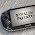3.Thanks for these questions. They were really very helpful !!!!!!!
Thanks again.....

4.thank u so much for these questions .they are very good .it made me understand this topic very well

1.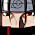yes... me 2.....

5.thank u very much... for giving these answers... :D

6.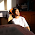thank you very much. it was very helpful

7.good work

8.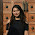Thank you so much.
It was really helpful ^_^

9.This comment has been removed by the author.

10.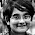Excellent ! This is the kind of stuff I have been searching for !!!

11.bakwas hai ncert ke 2 ya 3 hi question hai

12.Awesome!!! these answers were really very helpful!!!....

1.This comment has been removed by the author.

2.This comment has been removed by the author.

13.Thnx very much😊😎😎

14.Thnx very much😊😎😎

15.Really helped a lot!!!

16.Really helped a lot!!!

17.thank u it really helped a lot

18.love it ! thanks !

19.FANTASTC
SUMS!!!
THANKYOU for it!!!!!!!!!!!

20.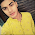Its fantastico... really helpfull..and thanxxxxx

21.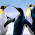15 th answer is wrong I hope so

22.Why don't you check it up
How will you take the speed as distance yaar

23.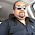24.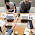25.Thanks.....my physics teacher is totally syco she gave us 150 numericals for our 20 days summer holidays.....I wanna f**king kill her

26.Thanks a Lot!!
Tmrw is my exam...this will help me a lot!

27.the answer of question 18 is wrong .It should be like this-
a=v-u/t
=22.2-16.6/5
=5.6/5
=1.12 m/s^2

28.now i have tension free from physics

29.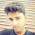these questions help me so much in exam , thanks a lot!!

30.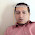31.This comment has been removed by the author.

32.33.34.This comment has been removed by the author.

35.36.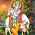Thanks a lot guys
Great work,,
Really